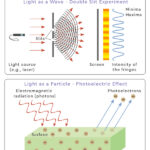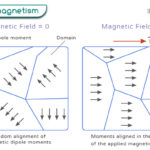Home / Physics / Thermal Radiation

Thermal radiation is defined as the transfer of heat in the form of electromagnetic waves. It lies in the infrared region of the electromagnetic spectrum. All substances above zero Kelvin emit thermal radiation. Photons carry the radiation energy. The photons travel until they are absorbed by another substance, making the latter feel warm.

Thermal radiation does not require any medium to propagate and can travel through a vacuum. This property makes thermal radiation unique compared to conduction and convection. However, it can be dangerous because the heating effect can cause burns and damage the skin.

## Examples

There are many sources of thermal radiation. Here are some examples:

• The Sun emits thermal radiation, which we perceive as warmth
• An electric stove emits thermal radiation, which cooks food
• A candle radiates heat. The color of its flame depends on the temperature

## Planck-Einstein Relation

The physical parameters that define a wave are wavelength (λ), frequency (ν), and speed (c). Planck’s equation gives the energy carried by the photon.

E = hν = hc/λ

Where h is known as Planck’s constant.

### Stefan-Boltzmann Law

Stefan-Boltzmann Law establishes a relationship between the radiative power and the temperature of the emitting surface. According to this law, the radiant heat energy emitted per second per unit area by a surface is proportional to the fourth power of its absolute temperature. Mathematically, this law can be written as follows:

P/A = σT4

Where

P: Power

A: Surface area

σ: Stephan-Boltzmann constant

T: Temperature

The radiative power depends on the nature of the emitting substance. Usually, blackened substances are good emitters and absorbers. A substance that absorbs all radiation falling in it is called a blackbody.

### Wien’s Law

Wien’s law or Wien’s displacement law is another law that can quantify thermal radiation. It determines the wavelength at which the radiation is at its peak. Mathematically, this law is given by

λmax T = 2.9 x 10-3 m·K

Where λ is the wavelength in meters, and T is the temperature in Kelvin. It can be seen that the maximum wavelength of radiation is inversely proportional to the temperature of the blackbody.

Thermal radiation gets its name because the amount of radiation, and its corresponding, spectrum depends on the temperature of the emitting body.

Article was last reviewed on Monday, January 2, 2023

### Related articlesWave-Particle DualityPhotoelectric EffectFerromagnetismDiamagnetism